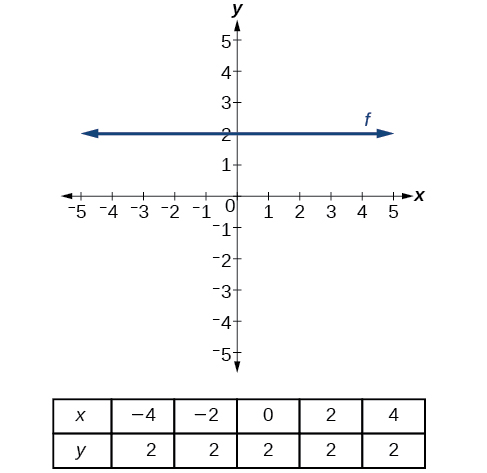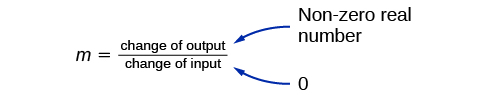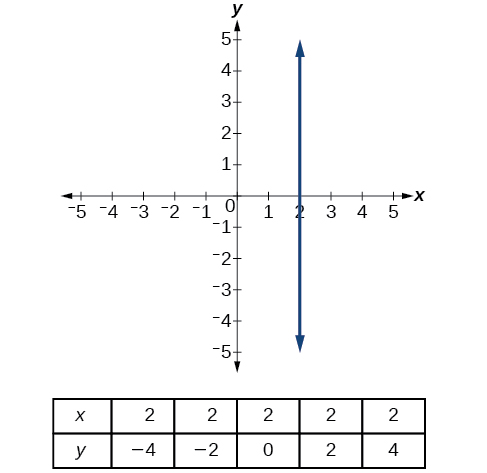# 4.1 Linear functions  (Page 11/27)

 Page 11 / 27A horizontal line representing the function   f ( x ) = 2

A vertical line    indicates a constant input, or x -value. We can see that the input value for every point on the line is 2, but the output value varies. Because this input value is mapped to more than one output value, a vertical line does not represent a function. Notice that between any two points, the change in the input values is zero. In the slope formula, the denominator will be zero, so the slope of a vertical line is undefined.Example of how a line has a vertical slope. 0 in the denominator of the slope.

A vertical line, such as the one in [link] , has an x -intercept, but no y- intercept unless it’s the line $\text{\hspace{0.17em}}x=0.\text{\hspace{0.17em}}$ This graph represents the line $\text{\hspace{0.17em}}x=2.$The vertical line,   x = 2 ,   which does not represent a function

## Horizontal and vertical lines

Lines can be horizontal or vertical.

A horizontal line    is a line defined by an equation in the form $\text{\hspace{0.17em}}f\left(x\right)=b.$

A vertical line    is a line defined by an equation in the form $\text{\hspace{0.17em}}x=a.$

## Writing the equation of a horizontal line

Write the equation of the line graphed in [link] .

For any x -value, the y -value is $\text{\hspace{0.17em}}-4,\text{\hspace{0.17em}}$ so the equation is $\text{\hspace{0.17em}}y=-4.$

## Writing the equation of a vertical line

Write the equation of the line graphed in [link] .

The constant x -value is $\text{\hspace{0.17em}}7,$ so the equation is $\text{\hspace{0.17em}}x=7.$

## Determining whether lines are parallel or perpendicular

The two lines in [link] are parallel lines    : they will never intersect. They have exactly the same steepness, which means their slopes are identical. The only difference between the two lines is the y -intercept. If we shifted one line vertically toward the other, they would become coincident.

We can determine from their equations whether two lines are parallel by comparing their slopes. If the slopes are the same and the y -intercepts are different, the lines are parallel. If the slopes are different, the lines are not parallel.

Unlike parallel lines, perpendicular lines    do intersect. Their intersection forms a right, or 90-degree, angle. The two lines in [link] are perpendicular.

Perpendicular lines do not have the same slope. The slopes of perpendicular lines are different from one another in a specific way. The slope of one line is the negative reciprocal of the slope of the other line. The product of a number and its reciprocal is $\text{\hspace{0.17em}}1.\text{\hspace{0.17em}}$ So, if are negative reciprocals of one another, they can be multiplied together to yield $\text{\hspace{0.17em}}–1.$

${m}_{1}{m}_{2}=-1$

To find the reciprocal of a number, divide 1 by the number. So the reciprocal of 8 is $\text{\hspace{0.17em}}\frac{1}{8},\text{\hspace{0.17em}}$ and the reciprocal of $\text{\hspace{0.17em}}\frac{1}{8}\text{\hspace{0.17em}}$ is 8. To find the negative reciprocal, first find the reciprocal and then change the sign.

As with parallel lines, we can determine whether two lines are perpendicular by comparing their slopes, assuming that the lines are neither horizontal nor vertical. The slope of each line below is the negative reciprocal of the other so the lines are perpendicular.

The product of the slopes is –1.

the third and the seventh terms of a G.P are 81 and 16, find the first and fifth terms.
if a=3, b =4 and c=5 find the six trigonometric value sin
pls how do I factorize x⁴+x³-7x²-x+6=0
in a function the input value is called
how do I test for values on the number line
if a=4 b=4 then a+b=
a+b+2ab
Kin
commulative principle
a+b= 4+4=8
Mimi
If a=4 and b=4 then we add the value of a and b i.e a+b=4+4=8.
Tariq
what are examples of natural number
an equation for the line that goes through the point (-1,12) and has a slope of 2,3
3y=-9x+25
Ishaq
show that the set of natural numberdoes not from agroup with addition or multiplication butit forms aseni group with respect toaaddition as well as multiplication
x^20+x^15+x^10+x^5/x^2+1
evaluate each algebraic expression. 2x+×_2 if ×=5
if the ratio of the root of ax+bx+c =0, show that (m+1)^2 ac =b^2m
By the definition, is such that 0!=1.why?
(1+cosA+IsinA)(1+cosB+isinB)/(cos@+isin@)(cos$+isin$)
hatdog
Mark
jaks
Ryan
how we can draw three triangles of distinctly different shapes. All the angles will be cutt off each triangle and placed side by side with vertices touching

#### Get Jobilize Job Search Mobile App in your pocket Now!ByByBy Brooke DelaneyBy Anh DaoBy OpenStaxBy Brooke DelaneyBy Brooke DelaneyBy Mistry BhaveshBy OpenStaxBy Jonathan LongBy Dionne MahaffeyBy OpenStax